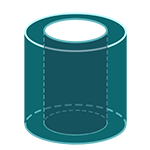Report a Problem
Suggestions

#Calculate The Volume of A Hollow Right Cylinder

Last updated: Saturday, April 29, 2023
Select a cylindrical shape below
Right Cylinder
Hollow Cylinder
Oblique Cylinder
Right Truncated
Cylindrical Wedge

A hollow right cylinder is a three-dimensional shape that consists of a cylinder with a hollow center. It has a circular base, straight parallel sides, and two circular faces at the top and bottom. The inner and outer radii of the cylinder can vary, but the height remains constant.

Some real-life examples of objects with similar shapes to a hollow right cylinder are a pipe, a thermos, and a cardboard roll from a roll of paper towels or toilet paper. These objects are used to contain fluids or other materials and protect them from the environment.

Easily calculate the volume of a hollow right cylinder with step-by-step guidance using our free calculator below.

The formula for determining the volume of a hollow right cylinder is defined as:
$$V$$ $$=$$ $$\pi$$ $$\cdot$$ $$h$$ $$\cdot$$ $$(R^2$$ $$-$$ $$r^2)$$
$$V$$: the volume of the hollow cylinder
$$r$$: the radius of the inner ring
$$R$$: the radius of the outer ring
$$h$$: the height of the cylinder
The SI unit of volume is: $$cubic \text{ } meter\text{ }(m^3)$$

## Find $$V$$

Use this calculator to determine the volume of a hollow right cylinder when the radii of the inner and outer ring bases and the height are given.
Hold & Drag
CLOSE
the radius of the inner ring
$$r$$
$$meter$$
the radius of the outer ring
$$R$$
$$meter$$
the height of the cylinder
$$h$$
$$meter$$
Bookmark this page or risk going on a digital treasure hunt again